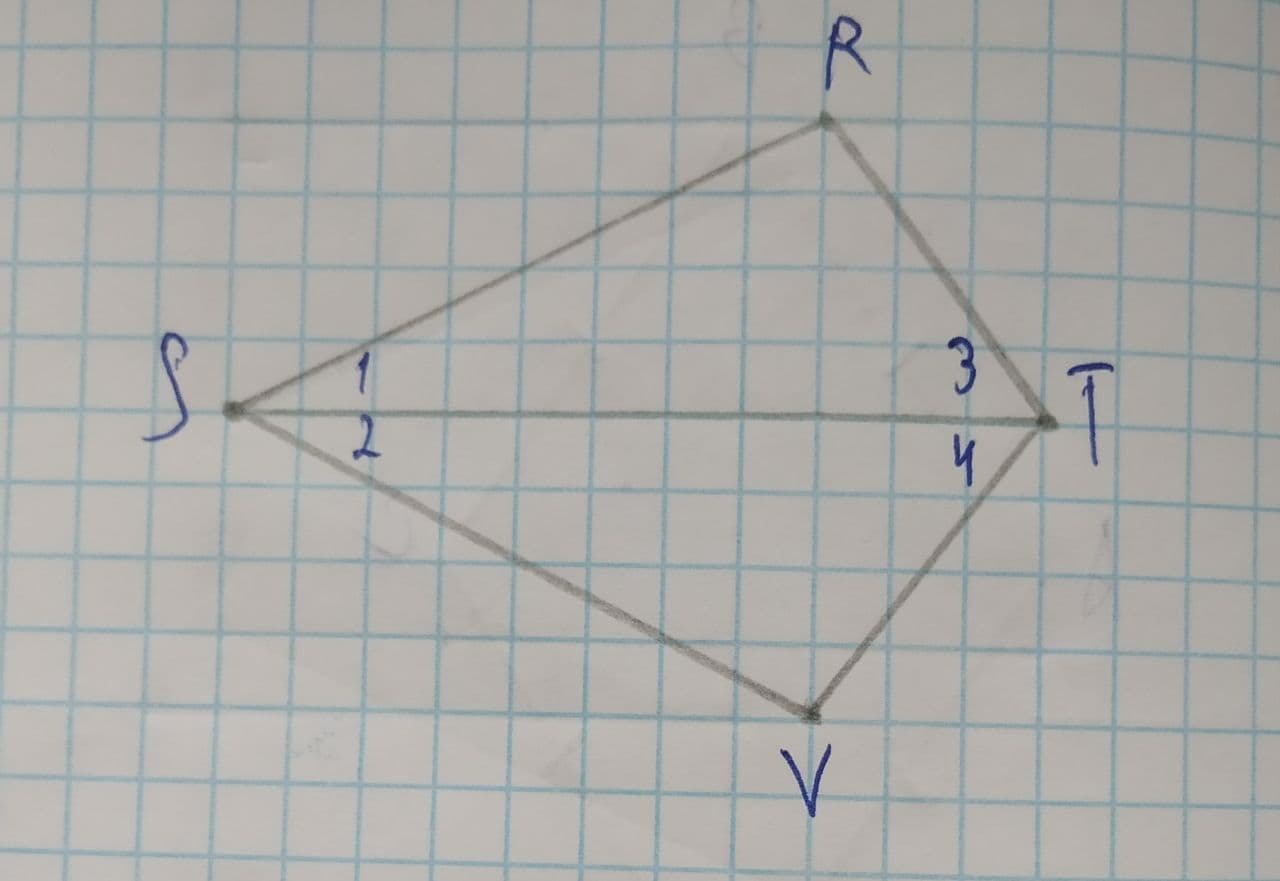Question# Complete all statements and reasons for the following proof problem \angle R and \angle V are right angles. \angle 1\cong\angle 2.

Congruence
ANSWEREDComplete all statements and reasons for the following proof problem.
Given:
$$\displaystyle\angle{R}$$ and $$\displaystyle\angle{V}$$ are right angles. $$\displaystyle\angle{1}\stackrel{\sim}{=}\angle{2}$$
Prove: $$\displaystyle\triangle{R}{S}{T}\stackrel{\sim}{=}\triangle{V}{S}{T}$$2021-07-26
Step 1
Given: $$\displaystyle\angle{R}=\angle{V}={90}^{{\circ}},\angle{1}\stackrel{\sim}{=}\angle{2}$$
For proving given thing, we take $$\displaystyle\triangle{R}{S}{T}\ {\quad\text{and}\quad}\ \triangle{V}{S}{T}$$
Step 2
So, $$\displaystyle\triangle{R}{S}{T}\ {\quad\text{and}\quad}\ \triangle{V}{S}{T}$$
$$\displaystyle\angle{R}=\angle{V}$$ (given)
$$\displaystyle\angle{1}=\angle{2}$$ (given)
ST=ST (common)
So, by ASA congruency rule
$$\displaystyle\triangle{R}{S}{T}\stackrel{\sim}{=}\triangle{V}{S}{T}$$
Hence, $$\displaystyle\triangle{R}{S}{T}\ {\quad\text{and}\quad}\ \triangle{V}{S}{T}$$ are congruence.